## Phasor

The representation, beloved of engineers and physicists, of a Complex Number in terms of a Complex exponential(1)

where i (called j by engineers) is the Imaginary Number and the Modulus and Argument (also called Phase) are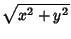(2)(3)

Here,is the counterclockwise Angle from the Positive Real axis. In the degenerate case when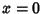,(4)

It is trivially true that(5)

Now consider a Scalar Function. Then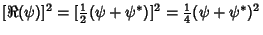(6)

Look at the time averages of each term,(7)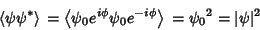(8)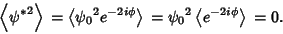(9)

Therefore,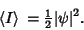(10)

Consider now two scalar functions(11)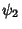(12)

Then(13)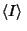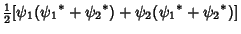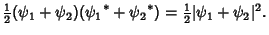(14)

In general,(15)

See also Affix, Argument (Complex Number), Complex Multiplication, Complex Number, Modulus (Complex Number), Phase